# MSO

## Monadic second-order logic with cardinality predicates ★★

Author(s): Courcelle

The problem concerns the extension of Monadic Second Order Logic (over a binary relation representing the edge relation) with the following atomic formulas:

\item\item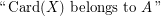where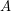is a fixed recursive set of integers.

Let us fixand a closed formula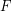in this language.

Conjecture   Is it true that the validity offor a graphof tree-width at mostcan be tested in polynomial time in the size of?

## Order-invariant queries ★★

Author(s): Segoufin

Question
\item Doeshold over graphs of bounded tree-width? \item Is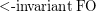included in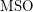over graphs? \item Doeshave a 0-1 law? \item Are properties ofHanf-local? \item Is there a logic (with an effective syntax) that captures?